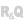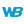HelpEditorsControls (window/page/report)ControlsTable controlMemory tableTable control populated programmatically: WLanguage (prefix syntax)OverviewInitializing a Table control populated programmaticallyAdding rows into a Table control populated programmaticallyManaging calculated columnsModifying a row or a columnOperations on the rows and cellsSee alsoTable control populated programmatically: WLanguageTable control populated programmatically: WLanguage (prefix syntax)The Table control populated programmatically
 OverviewInitializing a Table control populated programmaticallyAdding rows into a Table control populated programmaticallyManaging calculated columnsModifying a row or a columnForcing the inputAdding or deleting a columnOperations on the rows and cellsDeleting a rowRetrieving the content of a row or cellValue of a cell in the current rowValue of a cell in a specific rowContent of the current rowContent of a specific row WINDEVWEBDEVWINDEV MobileOthersTable control populated programmatically: WLanguage (prefix syntax) Overview Here is an overview of how to handle Table controls populated programmatically in WLanguage. WLanguage includes multiple functions to handle this type of control in the code. To learn more on these functions, see the documentation. For more details, see Table control management functions. Initializing a Table control populated programmatically Rows are not created automatically in a Table control. This must be specified by calling:.Add or .AddLine: adds a row at the end of the Table control. .Insert or .InsertLine: adds a row at the specified index or at the current index. Remark: if "Cascading input (Addition)" is not selected:The Table control contains no rows when it is created: TABLE_MyTable.Count = 0. In this case, no input is allowed and Empty is set to True.To automatically insert rows into a Table control, write the following lines of code in the control initialization event: IF TABLE_MyTable.Empty = True THEN TABLE_MyTableAdd() Adding rows into a Table control populated programmatically You can add rows to a Table control:with .AddLine and .InsertLine: in each row, the value of each column is separated by commas.with .Add and .Insert: in a row, the value of each column is separated by TAB.If a new row was added to the Table control with .Add, .Insert, .AddLine or .InsertLine, New is set to True (otherwise, it is set to False).Managing calculated columns The calculation formula of a calculated column must be defined in the "Display a row" event of a Table control. For example: // COL_PBT: calculated column// COL_UPBT and COL_QTY: columns populated programmaticallyCOL_PBT = COL_UPBT * COL_QTY Modifying a row or a column The content of the rows and columns in a Table control can be modified:by the user, by entering data directly in the columns. The changes are automatically saved in the Table control (no additional code is required). The Modified property is set to True.programmatically:with .Modify to change the content of the current row or the content of a given row.For example: TABLE_CUSTOMER.Modify("MOORE" + TAB + "Vince" + TAB + "Miami") Remark: you can also use the name of the Table control directly: // Modify the current rowTABLE_CUSTOMER = "MOORE" + TAB +"Vince" + TAB + "Miami"// Modify row 3TABLE_DAY.Modify("Wednesday" + TAB + "Off", 3) by specifying the name of the column to change the content (as for an Edit control). To modify the column of a specific row, the row number must be specified (index).For example: COL_NAME[Index] = EDT_CustomerName To modify the column of the current row, there is no need to specify the index. For example: COL_NAME = EDT_CustomerName The Modified property is set to False (it is set to True only when data is entered in the Table control).Forcing the inputSetFocusAndReturnToUserInput can be used to resume input in a column, on the current row.For example: // Entry process in the COL_QTY column// COL_QTY cannot be entered, // if COL_PRODUCT is not enteredIF NoSpace(COL_PRODUCT) = "" THEN Error("The Product column must be entered first") SetFocusAndReturnToUserInput(COL_PRODUCT)ENDAdding or deleting a columnYou can:Add a column to a Table control populated programmatically with ControlClone.Delete a column from a Table control populated programmatically with ControlDelete.Operations on the rows and cells Deleting a rowYou can delete rows by calling .Delete. The syntax used is as follows: .Delete([]) If the index is specified, .Delete deletes the row that corresponds to that index. Otherwise, the current row is deleted. For example: TABLE_CUSTOMER.Delete() A row can be selected with .SelectPlus.The syntax used is as follows: .SelectPlus([]) Retrieving the content of a row or cellThe content of a Table control populated programmatically can be retrieved:for the entire row. cell by cell.Value of a cell in the current rowTo retrieve the value of a column (or cell) for the current row, the syntax is the same as for a simple edit control. = Example: // COL_QTY is a column of the Table controlIF COL_QTY < 10 THEN Info("Insufficient quantity")END Value of a cell in a specific rowTo retrieve the value of a column that is not in the current row, you must specify the index of the row. = [] For example: // Add the total price before tax (PBT) for all the order linesTotalPrice = 0FOR Index = 1 _TO_ TABLE_ORDER.Count TotalPrice = TotalPrice + COL_PBT[Index]END Content of the current rowTo retrieve the content of the current row, use the following syntax: = Content of a specific rowTo get the content of the row at index , use the following syntax: = [] Remark: The index of the current row is returned by .Select. For example: // Retrieve row #10 in the TABLE_CUSTOMER controlCurrentRow = TABLE_CUSTOMER // Name of selected customerCustomerName = COL_NAME[TABLE_CUSTOMER.Select()] // Retrieve the current row in the TABLE_CUSTOMER controlCurrentRow = TABLE_CUSTOMER Minimum version required Version 23 This page is also available for…